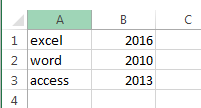# Swapping Two Columns or Rows in Excel

This post will guide you how to swap rows or columns in Excel. How do I swap cell values between rows or columns in Excel. How to swap columns or rows with VBA Macro in Excel. How to swap columns in Excel. How to swap two range of cells with VBA macro in Excel.

## Swap Two Columns

If you want to swap two ranges in different columns or rows in Excel, you can write down an Excel VBA Macro to quickly swap the selected two ranges. Just do the following steps:

#1 open your excel workbook and then click on “Visual Basic” command under DEVELOPER Tab, or just press “ALT+F11” shortcut.#2 then the “Visual Basic Editor” window will appear.

#3 click “Insert” ->”Module” to create a new module.#4 paste the below VBA code into the code window. Then clicking “Save” button.

```Sub SwapRange()
Dim Range1 As Range
Dim Range2 As Range
Set Range1 = Application.Selection
Set Range1 = Application.InputBox("select the first range:", "SwapRange", Range1.Address, Type:=8)
Set Range2 = Application.InputBox("select the second range:", "SwapRange", Type:=8)
tmp1 = Range1.Value
tmp2 = Range2.Value
Range1.Value = tmp2
Range2.Value = tmp1
End Sub```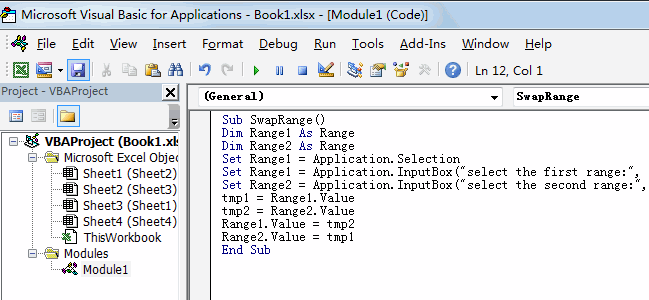#5 back to the current worksheet, then run the above excel macro. Click Run button.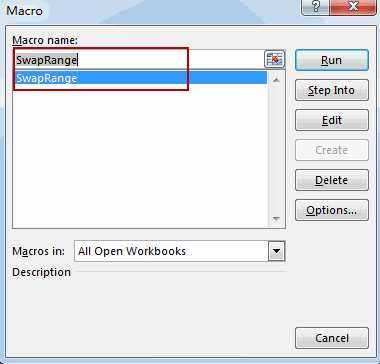#6 select the first range, such as: A1:A3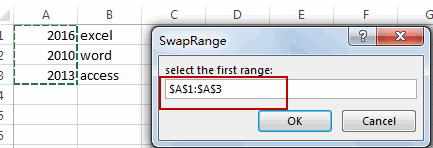#7 select the second range, such as: B1:B3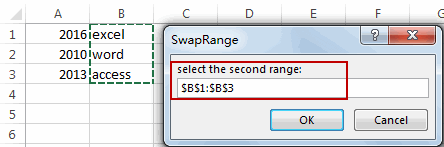#8 let’s see the result.